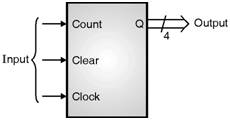Home > VHDL > Counters > Binary up counter

An n-bit binary counter can be constructed using a modified n-bit register where the data inputs for the register come from an incrementer (adder) for an up counter. Starting with a value stored in a register, to get to the next up count sequence, we simply have to add a one
to it.

A 4-bit binary up counter with asynchronous clear :

The behavioral VHDL code for the 4-bit binary up counter is shown in Fig. 2.13.1. The statement USE IEEE.STD_LOGIC_UNSIGNED.ALL is needed in order to perform additions on STD_LOGIC_VECTORs.library ieee;

use ieee.std_logic_1164.all;

use ieee.std_logic_unsigned.all; -- need this to add std_logic_vectors

entity counter is

port (clock: in std_logic;

clear: in std_logic;

count: in std_logic;

q : out std_logic_vector(3 downto 0)); -- no. of bits can be change in this line.

end counter;

architecture behav of counter is

signal value: std_logic_vector(3 downto 0); -- no. of bits can be change in this line

begin

process (clock, clear)

begin

if clear = '1' then

value <= (others => '0'); -- 4-bit vector of 0, same as "0000"

elsif (clock'event and clock='1') then

if count = '1' then

value <= value + 1; -- + sign for up count

end if;

end if;

end process;

q <= value;

end behav;